# Evaluating the Stolt stretch parameter

Sergey Fomel

sergey@sep.stanford.edu

# ABSTRACT

The Stolt migration extension to a varying velocity case (Stolt stretch) implies describing a vertical heterogeneity by a constant parameter (W). This paper exploits the connection between modified dispersion relations and traveltime approximations to derive an explicit expression for W. The expression provides theoretically the highest possible accuracy within the Stolt stretch framework. Applications considered include optimal partitioning of the velocity distribution for the cascaded migrations and extension of the Stolt stretch method to transversally isotropic models.

Stolt migration is regarded as the fastest post-stack migration method of all the known algorithms. A known price for that speed is the constant velocity assumption. The time-stretching trick proposed in Stolt's classic paper provides an approximate extension of the method to a variable velocity case. Stolt stretch implicitly transforms reflection traveltime curves to fit an approximate constant velocity pattern (, , ). In other words, the wave equation with variable velocity is transformed by a particular stretch of the time axis to an approximate differential equation with constant coefficients. The two constant coefficients are an arbitrarily chosen frame velocity and a specific nondimensional parameter (W in Stolt's original notation). In the constant velocity case W is equal to 1, and the transformed equation coincides with the exact constant velocity wave equation. In variable velocity media, W is generally assumed to lie between and 1. As shown by Beasley et al. , the cascaded f-k migration approach can move the value of W for each migration in a cascade closer to 1, thus increasing the accuracy of the Stolt stretch approximation.

The W factor was defined by Stolt as an approximate average of a complicated function. Stolt's definition cannot be used directly for computation because it includes a combined dependence on both time and space coordinates. Therefore, in practice, the estimation of this factor is always replaced by a heuristic guess. That's why Levin called the W parameter infamous'' (joking, of course), and Beasley et al. called it it esoteric.''

This paper develops a method to evaluate the Stolt stretch parameter explicitly. The main idea is to constrain the parameter by fitting the exact and approximated traveltime functions. In the case of isotropic interpretation, the W parameter is connected to the parameter of heterogeneity'' (, , ). In the case of anisotropic (transversally isotropic) interpretation, it can be related to the parameter of anellipticity'' (, ).

STOLT STRETCH THEORY In order to simplify the references, I will begin with the textbook definitions of the Stolt migration method. The reader familiar with Stolt stretch theory can skip this section and go on to a new piece of theory in the next one.

Post-stack seismic migration is theoretically a two-stage process consisting of wavefield downward continuation in depth z based on the wave equation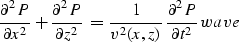(1)
and the imaging condition t=0 (here the velocity v is twice as small as the actual wave velocity). Stolt time migration performs both stages in one step, applying the frequency-domain operator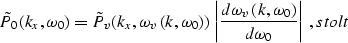(2)
where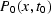stands for the initial zero-offset (stacked) seismic section defined on the surface z=0,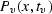is the time-migrated section, and tv is the vertical traveltime(3)
The functionin stolt corresponds to the dispersion relation of the wave equation wave and in the constant velocity case has the explicit expression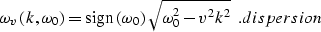(4)
The choice of the sign in dispersion is essential to distinguish between upgoing and downgoing waves. It is the upgoing part of the wave field that is used in migration.

For the case of a varying velocity Stolt suggested the following change of the time variable (referred to in the literature as Stolt stretch):(5)
where v0 is an arbitrarily chosen constant velocity, andis a function defined by the parametric expressions(6)
With the stretch ss, seismic time migration can be related to the transformed wave equation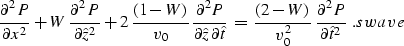(7)
Hereandare the transformed depth and time coordinates that possess the following property: if,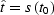, and if,. W is a varying coefficient defined as(8)
where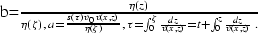Stolt's idea was to replace the slowly varying parameter W with its average value. Thus equation swave is approximated by an equation with constant coefficients, which has the dispersion relation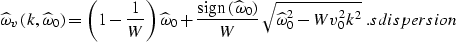(9)
Stolt's approximate method for migration in heterogeneous media consists of the following steps:

1.
stretching the time variable according to ss,
2.
interpolating the stretched time to a regular grid,
3.
double Fourier transform,
4.
f-k time migration by operator stolt with the dispersion relation sdispersion,
5.
inverse Fourier transform,
6.
inverse stretching (shrinking) the vertical time variable on the migrated section.
The value of W must be chosen prior to migration. According to Stolt's original definition wstolt, the depth variable z gradually changes in the migration process from zero to, causing the coefficient b in wstolt to change monotonically from 0 to 1. If the velocity v monotonically increases with depth, then, and the average value of b is(10)
As follows from wstolt and bishalf, in the case of monotonically increasing velocity, the average value of W has to be less than 1 (W equals 1 in a constant velocity case). Analogously, in the case of a monotonically decreasing velocity, W is always greater than 1. In practice, W is included in migration routines as a user-defined parameter, and its value is usually chosen to be somewhere in the range of 1/2 to 1.

In this paper I will describe a straightforward way to determine the most appropriate value of W for a given velocity distribution.

A useful tool for that purpose is Stewart Levin's formula for the traveltime curve. Levin applied the stationary phase technique to the dispersion relation sdispersion to obtain an explicit formula for the summation curve of the integral migration operator analogous to the Stolt stretch migration. The formula evaluates the summation path in the stretched coordinate system, as follows: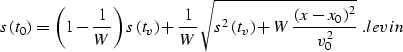(11)
Here x0 is the midpoint location on a zero-offset seismic section, and x is the space coordinate on the migrated section. Formula levin shows that, with the stretch of the time coordinate, the summation curve has the shape of a hyperbola with the apex at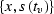and the center (the intersection of the asymptotes) at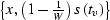. In the case of homogeneous media, W=1,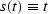, and levin reduces to the well-known hyperbolic diffraction traveltime curve. It is interesting to note that inverting formula levin for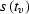determines the impulse response of the migration operator, which can be interpreted as the wavefront from a point source in thedomain of equation swave:(12)
where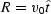, and Q=2-W. According to equation sfront, wavefronts from a point source in the stretched coordinates for W<2 have an elliptic shape, with the center of the ellipse at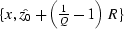and the semi-axesand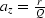. The ellipses stretch differently for W<1 and W>1 (Figure stofro). In the upper part that corresponds to the upgoing waves, they look nearly spherical, since the radius of the front curvature at the top apex equals the distance from the source.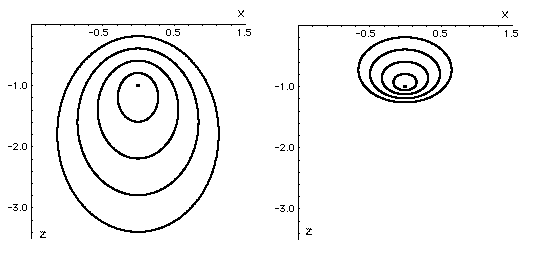stofro
Figure 1
Wavefronts from a point source in the stretched coordinate system. Left: velocity decreases with depth (W=1.5). Right: velocity increases with depth (W=0.5).EVALUATING THE W PARAMETER AND STOLT STRETCH ACCURACY

Formula levin belongs to the three-parameter class of traveltime approximations. The key result of this paper uses a remarkable formal similarity between levin and Malovichko's approximation for the reflection traveltime curve in vertically inhomogeneous media (, , ) defined by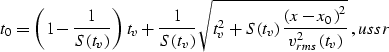(13)
where vrms stands for the effective (root mean square) velocity along the vertical ray(14)
and S is the parameter of heterogeneity: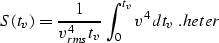(15)
In terms of the S parameter, the variance of the squared velocity distribution along the vertical ray is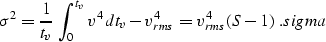(16)
As follows from equality sigma,for any type of velocity distribution (S equals 1 in a constant velocity case). For most of the distributions occurring in practice, S ranges between 1 and 2.

Malovichko's formula ussr is known as the most accurate three-parameter approximation of the NMO curve in vertically inhomogeneous media. Since reflection from a horizontal reflector in that class of media is kinematically equivalent to diffraction from a point, formula ussr can be similarly regarded as an approximation of the summation path of the post-stack Kirchhoff-type migration operator. In this case, it has the same meaning as formula levin. An important difference between the two formulae is the fact that equation ussr is written in the initial coordinate system and includes coefficients varying with depth, while equation levin applies the transformed coordinate system and constant coefficients. Using this fact, the rest of this section compares the accuracy of the approximations and relates Stolt's W factor to Malovichko's parameter of heterogeneity.

Equations levin and ussr both approximate the traveltime curve in the neighborhood of the vertical ray. Therefore, to compare their accuracy, it is appropriate to consider series expansion of the diffraction traveltime in the vicinity of the vertical ray: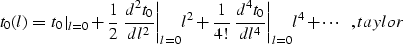(17)
where l=x-x0. Expansion taylor contains only even powers of l because of the obvious symmetry of t0 as a function of l.

The special choice of parameters tv, vrms, and S allows Malovichko's formula ussr to provide correct values for the first three terms of expansion taylor: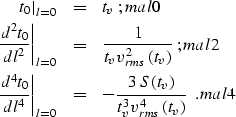(18) (19) (20)
Considering Levin's formula levin as an implicit definition of the function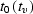, we can iteratively differentiate it following the rules of calculus: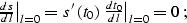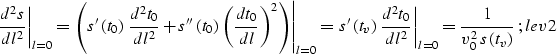(21)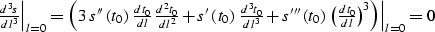(22)
Substituting the definition of Stolt stretch transform ss into lev2 produces an equality similar to mal2, which means that approximation levin is theoretically accurate in depth-varying velocity media up to the second term in taylor. It is this remarkable property that proves the validity of the Stolt stretch method (, ). Formula levin will be accurate up to the third term if the value of the fourth-order traveltime derivative in lev4 coincides with mal4. Substituting equation mal4 into lev4 transforms the latter to the form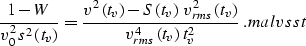(23)
It is now easy to derive from equation malvsst the desired explicit expression for the Stolt stretch parameter W, as follows: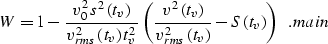(24)

Expression main is derived so as to provide the best possible value of W for a given depth (vertical time tv). To get a constant value for a range of depths one should take an average of the right hand side of main in that range. The error associated with Stolt stretch can be approximately estimated from taylor as the difference between the fourth-order terms: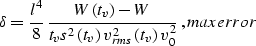(25)
where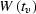is the right-hand side of main, and W is the constant value of W chosen for Stolt migration.

To estimate the best possible accuracy that the Stolt stretch method can achieve, we must take into account the sixth-order term in taylor related to the sixth-order derivative of the traveltime curve. For the true traveltime curve, the expression for the sixth-order derivative in the vicinity of the vertical ray is known from the literature (, ) to be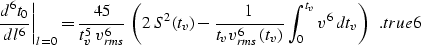(26)
First, let us estimate the error of Malovichko's approximation ussr. Differentiating ussr six times and setting the offset l to zero yields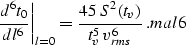(27)
The estimated error is proportional to the difference between mal6 and true6: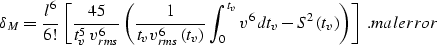(28)
It is interesting to note that replacing the parameter of heterogeneity S by its definition heter changes the expression in the round brackets to the following form: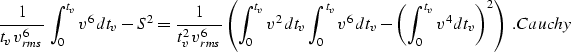(29)
According to the Schwarz inequality from calculus ( also known as the Cauchy-Bunyakovski inequality), the value of expression Cauchy can never be less than zero; hence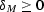for any velocity distribution. This conclusion indicates that Malovichko's approximation tends to increase the traveltime at large offsets beyond its true value.

Differentiating lev4 twice and eliminating terms that vanish at l=0 produces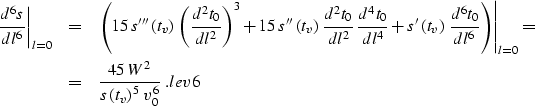(30)
Evaluating the sixth-order traveltime derivative from lev6 and subtracting true6, we get a somewhat lengthy but explicit expression for the error associated with Stolt stretch approximation in the case of the best possible choice of W:(31)

ISOTROPIC HETEROGENEITY VERSUS ANELLIPTIC ANISOTROPY A controversial issue associated with the topic of this paper is whether the non-hyperbolicity of the traveltime curves is caused mainly by heterogeneity or by anisotropy. To find a connection between the two different descriptions of media, we can consider an alternative three-parameter traveltime approximation (the anelliptic anisotropic moveout formula), proposed by Muir and Dellinger :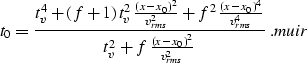(32)
Here f is the parameter of anellipticity. Differentiating muir four times, setting l=x-x0 to zero, and equating the result with mal4 results in the following formal relationship between f and Malovichko's parameter of heterogeneity: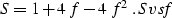(33)
Equation Svsf clearly demonstrates the uncertainty between the anisotropic and heterogeneous isotropic interpretations. Both of them can explain the cause of the nonhyperbolicity of traveltime curves. An important difference is that the parameter of heterogeneity is uniquely determined by the velocity distribution according to heter, while the f parameter is assumed to be an independent functional. The definition heter, applied in combination with main, is suitable for calculating the Stolt stretch factor in an isotropic model for a given velocity function. If the correction parameter is measured experimentally by a non-hyperbolic velocity analysis in the form of either equation ussr or equation muir, it accumulates both heterogeneous and anisotropic factors and can be used for an explicit determination of W in main independently of the preferred explanation. In the case of the anisotropic moveout velocity analysis, we merely need to substitute the connection formula Svsf into main to find W. An alternative approach to Stolt-type migration in transversally isotropic media was proposed recently by Ecker and Muir . However, Stolt stretch migration is superior to that method in its ability to cope with varying rms velocities.

EXAMPLES A simple analytic example of isotropic heterogeneity is the case of a constant velocity gradient. In this case the velocity distribution can be described by the linear function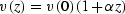. Stolt stretch transform is found from ss as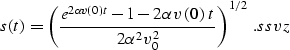(34)
Letbe the logarithm of the velocity change v(z)/v(0). Then an explicit expression for W factor follows from main:(35)
For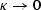(a small depth or a small velocity gradient),. For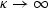(a large positive change of velocity) W monotonically approaches zero. Formula wvz can be a useful rule of thumb for a rough estimation of W.

Numerical example of the Stolt stretch parameter computation is illustrated in Figures stovwt and stocvw. The left side of Figure stovwt shows a smoothed interval velocity curve from the Gulf of Mexico. The corresponding optimal values of the W factor as a function of vertical time (in the isotropic model) are shown on the right. Though the velocity function is smooth, substantial changes in W occur, making its mean value for the timessec equal to 0.631.

The theory of cascaded migrations (, ) proves that Stolt-type f-k migration for a nonuniform velocity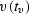can be performed as a cascaded process consisting of migrations with the smaller velocities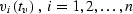, such that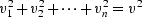. As shown by Larner and Beasley , it is important to partition the velocity so that for each particular tv all the velocities in the cascade, except maybe the last one, are constant. The advantage of the cascaded f-k migration method is based on the fact that each small velocity vi describes a more homogeneous medium than the initialfunction. Therefore, the W factor for each migration in a cascade is closer to 1, and the Stolt stretch approximation is more accurate. This fact is illustrated in Figure stocvw, which shows an optimal partitioning of the velocity and the corresponding values of the W factor. In accordance with the empirical conclusions of Beasley et al. , a cascade of only four migrations was sufficient to increase the value of W to more than 0.8. With a further increase of the number of cascaded migrations, the method becomes as accurate with respect to vertical velocity variations as phase-shift migration. Theoretically, this limit corresponds to the velocity continuation concept (). Note that the theory of cascaded f-k migration is strictly valid for isotropic models. The anisotropic interpretation does not support it, since the intrinsic anisotropy factor is not supposed to change with the velocity partitioning.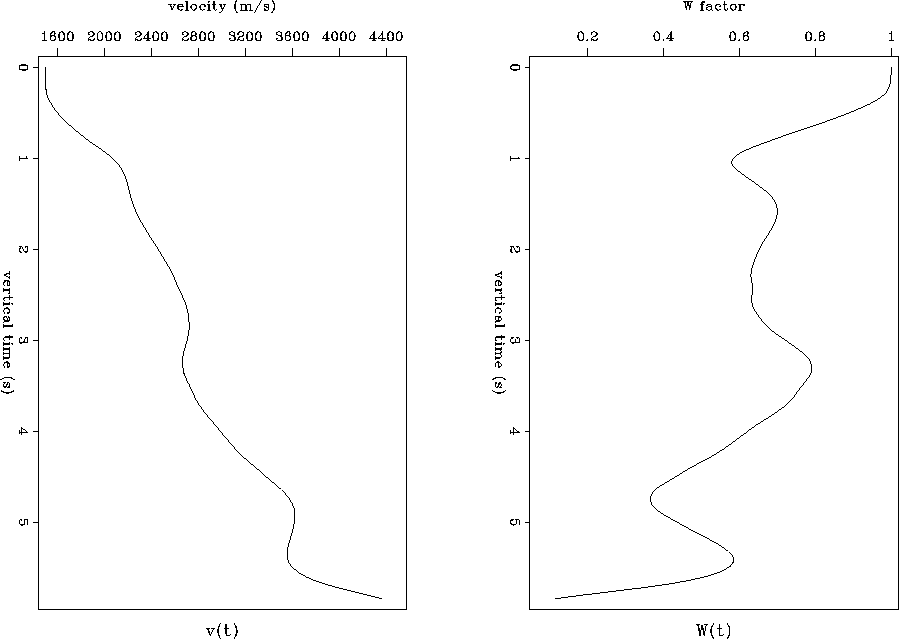stovwt
Figure 2
Smoothed interval velocity distribution from the Gulf of Mexico (left) and the corresponding W factor as a function of vertical time (right). The mean value of W is 0.631.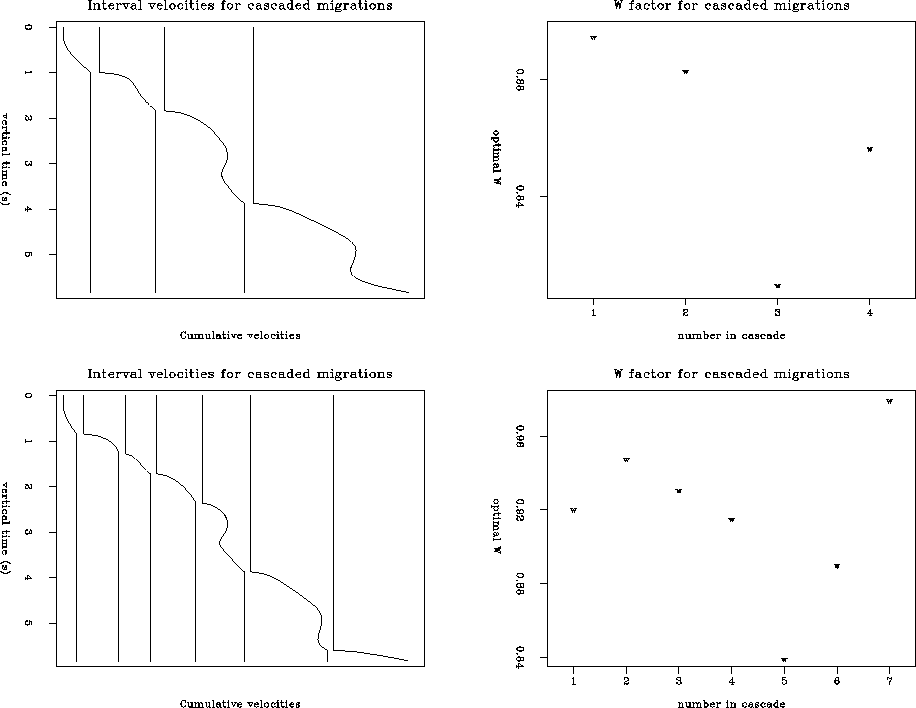stocvw
Figure 3
Left: Optimal partitioning of the velocity function for the method of cascaded migrations. Right: corresponding mean values of W. Top: four cascaded migrations. Bottom: seven cascaded migrations.

The main result of this paper is an analytic explicit expression main that allows us to choose the most appropriate value for the Stolt stretch factor. Possible applications include the optimal design of interval velocities partitioning for the method of cascaded f-k migrations and extension of the Stolt stretch method to a transversally isotropic model.

Nowadays the topic of this paper seems to be out of fashion. When everyone is interested in prestack depth migration in the time-space domain, it is difficult to attract any attention to post-stack time migration in the frequency domain. Nevertheless, I believe the art of approximation demonstrated by Robert Stolt in his famous paper to be a good example to follow when working on many different problems, which was the main reason for this research.

[SEP,MISC,GEOTLE,paper,SEGCON]